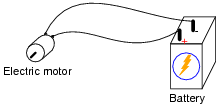# Elementary Circuits

## Basic Electricity

• #### Question 1

In the simplest terms you can think of, define what an electrical circuit is.

• #### Question 2

Given a battery and a light bulb, show how you would connect these two devices together with wire so as to energize the light bulb: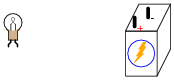• #### Question 3

Draw an electrical schematic diagram of a circuit where a battery provides electrical energy to a light bulb.

• #### Question 4

How could a battery, a light bulb, and some lengths of metal wire be used as a conductivity tester, to test the ability of different objects to conduct electricity?

• #### Question 5

Suppose we had a long length of electrical cable (flexible tubing containing multiple wires) that we suspected had some broken wires in it. Design a simple testing circuit that could be used to check each of the cable’s wires individually.• #### Question 6

What do the symbols with the question marks next to them refer to? In the circuit shown, would the light bulb be energized?• #### Question 7

Shown here is a simplified representation of an electrical power plant and a house, with the source of electricity shown as a battery, and the only electrical “load” in the house being a single light bulb: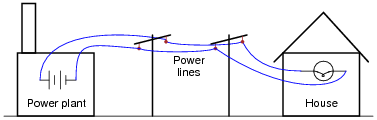Why would anyone use two wires to conduct electricity from a power plant to a house, as shown, when they could simply use one wire and a pair of ground connections, like this?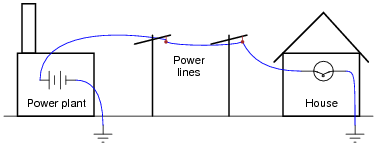• #### Question 8

What, exactly, is a short circuit? What does it mean if a circuit becomes shorted? How does this differ from an open circuit?

• #### Question 9

What would have to happen in this circuit for it to become shorted? In other words, determine how to make a short circuit using the components shown here: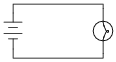• #### Question 10

Examine this schematic diagram:Now, without moving the following components, show how they may be connected together with wires to form the same circuit depicted in the schematic diagram above: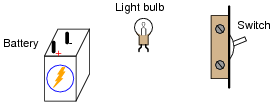• #### Question 11This circuit has four “test points” labeled with the letters A, B, C, and D. Assuming the circuit is functioning (light bulb is energized), determine whether or not there will be substantial voltage between the following sets of points:

• Between A and B: voltage or no voltage?
• Between B and C: voltage or no voltage?
• Between C and D: voltage or no voltage?
• Between D and A: voltage or no voltage?
• Between A and C: voltage or no voltage?
• Between D and B: voltage or no voltage?

Based on these voltage determinations, what general statement(s) can you make about the presence or absence of voltage in a functioning circuit?

• #### Question 12

In this electrical circuit, trace the direction of current through the wires: